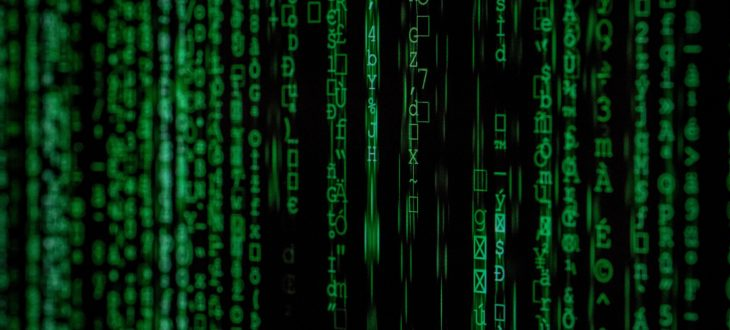# MRP 102 – BIOSTATISTICSCoordinator: George Sakellaropoulos

Lecturers: Sofia Daskalaki, Polychronis Economou, Sonia Malefaki and George Sakellaropoulos

Aim and Objectives

The course aims to provide the background of basic statistical concepts and measures, as well as major analytical statistical methodologies (tests), to support reasoning in biomedical science. Specific objectives include comprehension of descriptive statistics, formulation of the null hypothesis to support statistical reasoning, choice of the appropriate test for the problem at hand and critical assessment of test results.

Course Content

• Introduction: Basic concepts in probability, frequency and cumulative frequency, measures of central tendency and dispersion, conditional probability, diagnostic tests, definition of sensitivity, specificity and predictive value, Bayes theorem, receiver operating characteristic (ROC) analysis
• Random variables, probability distributions (Binomial, Poisson, Exponential, Normal), multivariate random variables, independence, covariance and correlation, conditional expectation and variance.
• Sampling Distributions: Central limit theorem, sampling error rate, sampling error of the difference between two random variables, standard error of the mean
• Parameter estimation: Method of moments, maximum likelihood method
• Statistical Inference – Interval Estimation: Introduction to interval estimation, confidence interval, prediction intervals, tolerance Intervals for several parameters of one or two populations.
• Statistical Inference – Hypothesis Testing: Introduction to hypothesis testing, type I and type II errors, p-value, power of a test and its relationship with the sample size, hypothesis tests for several parameters of one or two populations
• Analysis of Variance (ANOVA): Sum of squares (within, between, total), F-test
• Linear Regression and correlation: Simple linear regression model, fitting the least squares equation, analysis of Variance in regression, evaluation of the regression model, statistical inference on the model, regression with SPSS, linear correlation coefficient, conceptual difference between regression and correlation.
• Multiple Linear Regression: Fitting a multiple regression equation, analysis of variance in multiple regression, evaluation of the model, statistical Inference, choosing the right model, sequential methods for model selection
• Nonparametric Tests: Contingency tables, chi-square test, goodness-of-fit test, test of independence, test of homogeneity, test for several proportions, sign test, Wilcoxon signed-rank test, Kruskal-Wallis test, run test
• Survival Analysis: Time to event data, censored data, truncation, survival function, hazard function, cumulative hazard function, mean residual lifetime, estimation of the survival function (parametric and non-parametric models and methods)

In addition to lectures, the course aims to develop skills in the use of software for statistical analysis of medical and biological data (SPSS, Microsoft Excel, Graphpad Prism), through tutorials.

Suggested Textbooks

• Bland M, An Introduction to Medical Statistics, Oxford Medical Publications 2000
• Walpole-Myers, Probability & Statistics for engineers & scientists (9th edition), 2012
• Daniel W, Cross C, Biostatistics: A foundation for analysis in the Health Sciences (10th edition), 2013
• Vidakovic B, Engineering Biostatistics, 2016

Grading Policy: Mid-term & final written examination.